Courses

# Chapter 4 Measurement Of Power - Notes, Electrical Measurement, Electrical Engineering Electrical Engineering (EE) Notes | EduRev

## Electrical Engineering (EE) : Chapter 4 Measurement Of Power - Notes, Electrical Measurement, Electrical Engineering Electrical Engineering (EE) Notes | EduRev

The document Chapter 4 Measurement Of Power - Notes, Electrical Measurement, Electrical Engineering Electrical Engineering (EE) Notes | EduRev is a part of the Electrical Engineering (EE) Course Electrical Engineering SSC JE (Technical).
All you need of Electrical Engineering (EE) at this link: Electrical Engineering (EE)

Measurement Of Power

• Power Measurement in A.C. Circuit by Wattmeter Method :
• 3 general types of wattmeters are :
• Dynamometer type
• Induction type
• Electrostatic type
• Average power over a cycle in an AC circuits in given by,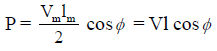where,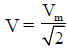= RMS value of voltage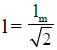= RMS value of current

and cosø = Power factor of the load

Elctrodynamometer Type Wattmeter :

• It consists of two coils, the “Fixed coil” or “Field coils” or “Current coils” (C.C) are connected in series with the load.

And the other coils is “Moving coils” or “Pressure coil” or “Voltage coil” or “Pontential coil (PC)“.

• The CC is made up of thick conductor because it has to carry the higher load current.
• The PC is made up thin conductor because it is designed to carry low load current.
• Spring control is used in this instrumment.
• Air friction damping is used.

Let rp and Lp are the resistance and inductance of the pressure coil or moving coil respectively and Rp = (rp + R) = total resistance of PC circuit

l = current in CC circuit = lCC
Since,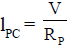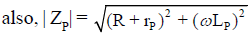= impedance of PC circuit.

Let load p.f. cosø lagging i.e. lags behind V by an angle ø .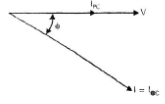• Deflecting torque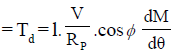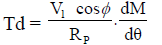and controlling torque = Tc = K θ

At null deflection, Tc = Td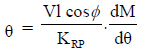since, θ ∝ Vl cos ø i.e.

θ ∝ Power (P)

• It indicates the uniform scale.

Measurement of 3-Phase Power with two wattmeter method :

• In a three phase, three wire system , we require 3-elements, but if we make the common points of the pressure coils coincide with one of the lines, then we will require only, n – 1 = 3 – 1 = 2, elements.
• Instantaneous power consumed by load is given by, p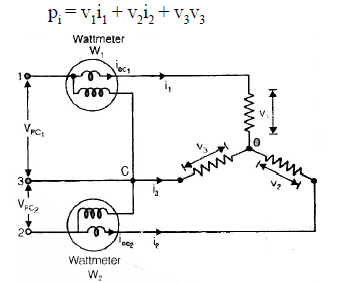• Let us consider two wattmeters connected to measure power in three phases circuit as shown in figure (star connection). Instantaneous reading of W1 wattmeter

P1 = i1(v1 – v3)

P2 = i2(v2 – v3)

Sum of instantaneous reading of two wattmeter is, P = P1 + P2

P = i1(v1 – v3) + i2(v2 – v3)

From KCL at node ‘O’, i1 + i2 + i3 = O, or (i1 + i2) = – i3

∴ sum of instantaneous readings of two wattmeters P = v1i1 + v2i2 + v3i3

• Therefore, the sum of the two wattmeter reading is equal to the power consumed by the load.
• This method is irrespective of whether the load is balanced or unbalanced.
• Observation :
• The phasor diagram for a balanced star connected load as shown in figure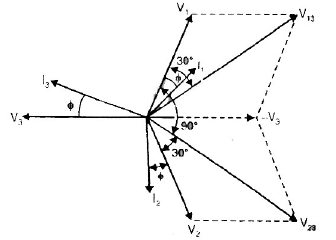Let V1, V2, V3, be the rms values of phase voltage and I1, I2, I3 be the rms values of phase currents.

Since the load is balanced, therefore,

Phase voltages, V1 = V2 = V3 = V(say)

Line voltages,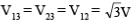Phase currents, I1 = I2 = I3 = I(say)

and, Power factor = cos ø

•  Actually phase currents and line currents both are same in star-connection. then reading of wattmeter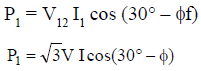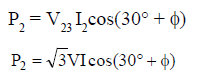Sum of reading of two wattmeter is, P = P1 + P2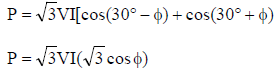P = 3 VI cosø = 3 - ø power
This shows the total power consumed by the load.

Since,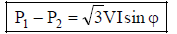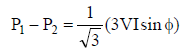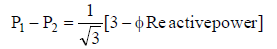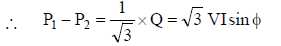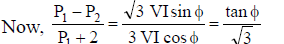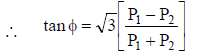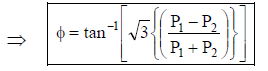From this equation (4.38) we have also find pf = cosø.

Offer running on EduRev: Apply code STAYHOME200 to get INR 200 off on our premium plan EduRev Infinity!

65 docs|37 tests

,

,

,

,

,

,

,

,

,

,

,

,

,

,

,

,

,

,

,

,

,

,

,

,

,

,

,

;